Gauge your potential with Aakash BYJU'S JEE Main'23 Mock Test Gauge your potential with Aakash BYJU'S JEE Main'23 Mock Test

# JEE Main 2021 March 17 – Shift 2 Chemistry Question Paper with Solutions

JEE Main 2021 March 17th Shift 2 Chemistry question paper with solutions are available here. Students are recommended to go through and revise these solutions so that they can be more familiar with the concepts as well as the overall pattern of the JEE Main question paper. Students can download the JEE Main March 17th question paper with solution as a PDF given below(available soon).

### JEE Main 2021 March 17th Shift 2 Chemistry Question Paper

SECTION-A

Question 1. Match List-I with List-II.

 List – I List – II Chemical Compound Used as (a) Sucralose (i) Synthetic detergent (b) Glyceryl ester of stearic acid (ii) Artificial sweetener (c) Sodium benzoate (iii) Antiseptic (d) Bithionol (iv) Food preservative

Choose the correct match:

1. a. (a)-(i), (b)-(ii), (c)-(iv), (d)-(iii)
2. b. (a)-(ii), (b)-(i), (c)-(iv), (d)-(iii)
3. c. (a)-(iv), (b)-(iii), (c)-(ii), (d)-(i)
4. d. (a)-(iii), (b)-(ii), (c)-(iv), (d)-(i)

Solution:

(a) Sucralose → Artificial sweetener

(b) Glyceryl ester of stearic acid → Synthetic detergent

(c) Sodium benzoate → Food preservative

(d) Bithionol → Antiseptic

Question 2. In the below reactions, enzyme A and enzyme B respectively are: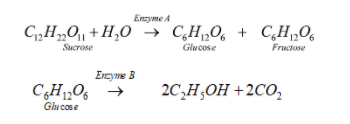1. a. Invertase and Amylase
2. b. Amylase and Invertase
3. c. Invertase and Zymase
4. d. Zymase and Invertase

Solution: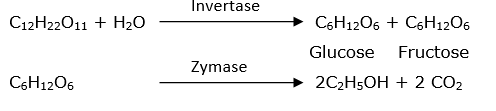Question 3. The correct pair(s) of the ambident nucleophiles is (are):

(A) AgCN/KCN

(B) RCOOAg/RCOOK

(C) AgNO2/KNO2

(D) AgI/KI

1. a. (A) and (C) only
2. b. (B) only
3. c. (B) and (C) only
4. d. (A) only

Solution: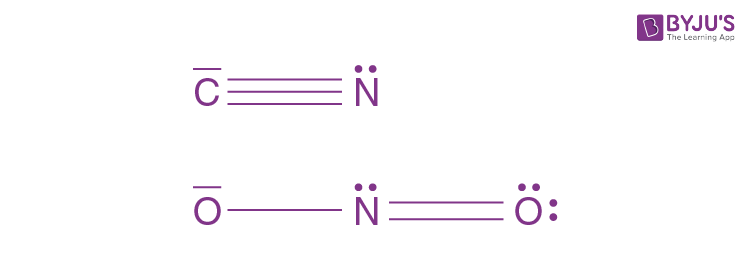More than one electron-donating side

Question 4. During which of the following processes, does entropy decrease?

(A) Freezing of water to ice at 0°C

(B) Freezing of water to ice at -10°C

(C) N2(g) + 3H2(g) →2NH3(g)

(E) Dissolution of NaCI in water

1. a. (A), (B), (C) and (D) only
2. b. (A), (C) and (E) only
3. c. (A) and (E) only
4. d. (B) and (C) only

Solution:

A, B → Freezing of water will decrease entropy as particles will move closer and forces of attraction will increase. This leads to a decrease in randomness. So, entropy decreases.

C → No. of molecules decreasing

D → Adsorption will lead to a decrease in the randomness of gaseous particles.

E → NaCl(s) → Na+ (aq) + Cl(aq); ΔS > 0

So, (A, B, C, D) decreases entropy.

Question 5. Match List-I with List-II:

 List – I List – II (a) [Co(NH3)6] [Cr(CN)6] (i) Linkage isomerism (b) [Co(NH3)3 (NO2)3] (ii) Solvate isomerism (c) [Cr(H2O)6]Cl3 (iii) Coordination isomerism (d) cis-[CrCl2(ox)2]3- (iv) Optical isomerism

Choose the correct answer from the options given below:

1. a. (a)-(iii), (b)-(i), (c)-(ii), (d)-(iv)
2. b. (a)-(i), (b)-(ii), (c)-(iii), (d)-(iv)
3. c. (a)-(ii), (b)-(i), (c)-(iii), (d)-(iv)
4. d. (a)-(iv), (b)-(ii), (c)-(iii), (d)-(i)

Solution:

Theory-based

Question 6. The common positive oxidation states for an element with atomic number 24, are

1. a. +1 and +3
2. b. +1 to +6
3. c. +1 and +3 to +6
4. d. +2 to +6

Solution:

Fact

Question 7. The set of elements that differ in a mutual relationship from those of the other sets is:

1. a. Be – Al
2. b. Li – Na
3. c. B – Si
4. d. Li – Mg

Solution:

Li and Na do not have a diagonal relationship.

Question 8. Given below are two statements:

Statement I: 2-methyl butane on oxidation with KMnO4 gives 2-methyl butane-2-ol.

Statement II: n-alkanes can be easily oxidized to corresponding alcohols with KMnO4.

Choose the correct option:

1. a. Both statement I and statement II are incorrect
2. b. Statement I is correct but statement II is incorrect
3. c. Both statement I and statement II are correct
4. d. Statement I is incorrect but statement II is correct

Solution: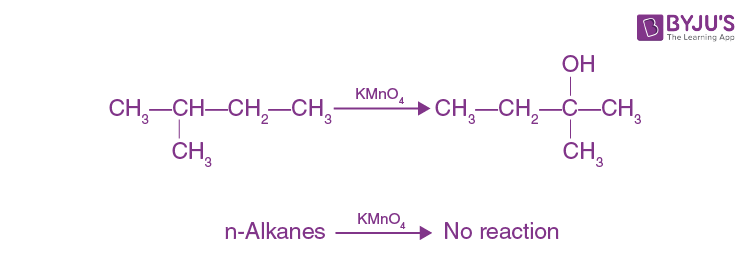Question 9. Amongst the following, the linear species is:

1. a. N-3
2. b. Cl2O
3. c. O3
4. d. NO2

Solution:

(a)

$$\bar{N} = \overset{+}{N} = \bar{N}$$
sp (linear)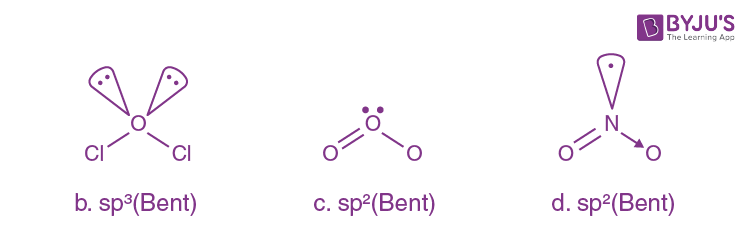Question 10. For the coagulation of a negative sol, the species below, that has the highest flocculating power is:

1. a. SO2-4
2. b. Na+
3. c. Ba2+
4. d. PO34

Solution:

For a negative sol, a positive ion is required for flocculation.

Greater the valence of the flocculating ion added, the greater is its power to cause precipitation.

This is called Hardy-Schulz law.

So, Ba+2 has the highest flocculating power.

Question 11. The functional groups that are responsible for the ion-exchange property of cation and anion exchange resins, respectively, are:

1. a. –SO3H and –COOH
2. b. –SO3H and –NH2
3. c. –NH2 and –SO3H
4. d. –NH2 and –COOH

Solution:

–SO3H and –COOH are cation exchange resin and –NH2 is anion exchange resin.

Question 12. Choose the correct statement regarding the formation of carbocations A and B given.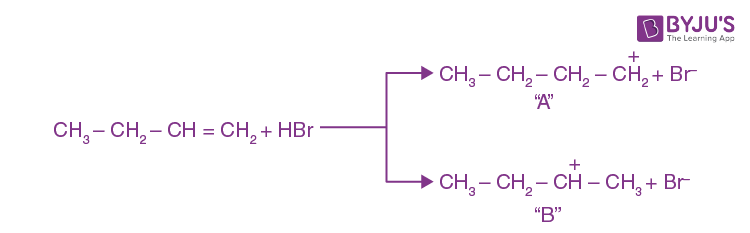1. a. Carbocation A is more stable and formed relatively at a faster rate
2. b. Carbocation B is more stable and formed relatively at a faster rate
3. c. Carbocation A is more stable and formed relatively at a slow rate
4. d. Carbocation B is more stable and formed relatively at a slow rate

Solution:

B carbocation is more stable due to more hyperconjugation and it forms a relatively faster rate compared to A.

Question 13. In the below reaction, the structural formula of (A), “X” and “Y” respectively are: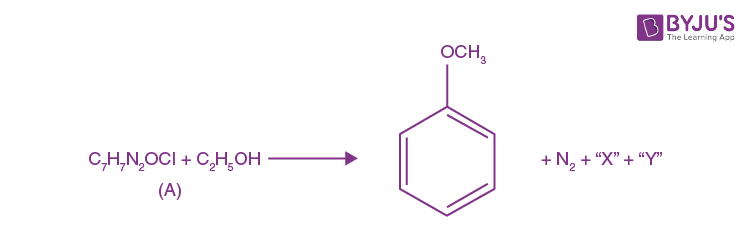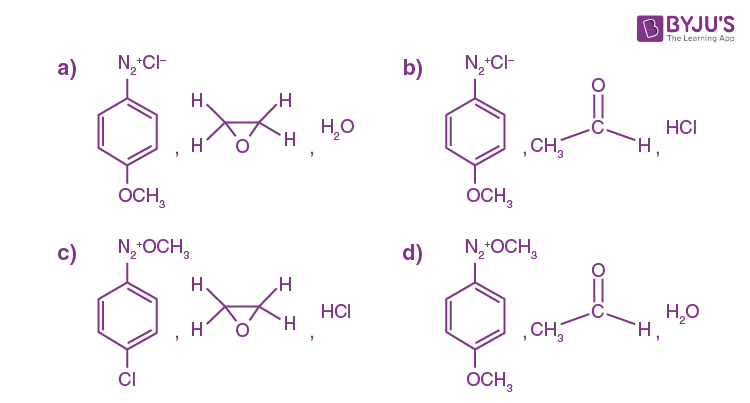Solution: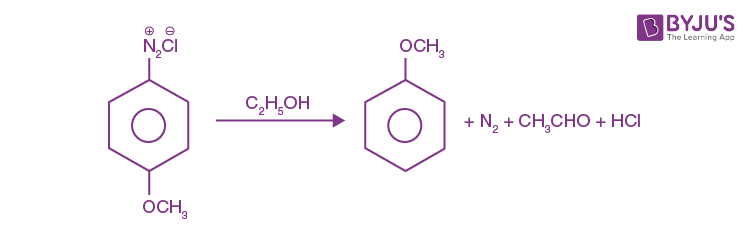Question 14. Fructose is an example of:

1. a. Heptose
2. b. Aldohexose
3. c. Pyranose
4. d. Ketohexose

Solution:

Fructose is an example of Ketohexose.

Question 15. Which of the following statement(s) is (are) the incorrect reason for eutrophication?

(A) excess usage of fertilisers

(B) excess usage of detergents

(C) dense plant population in water bodies

(D) lack of nutrients in water bodies that prevent plant growth

Choose the most appropriate answer from the option given below:

1. a. (D) only
2. b. (C) only
3. c. (B) and (D) only
4. d. (A) only

Solution:

Lack of nutrients in water bodies that prevent plant growth.

Question 16. Primary, secondary and tertiary amines can be separated using:

1. a. Para-Toluene sulphonyl chloride b. Chloroform and KOH c. Acetyl amide d. Benzene sulphonic acid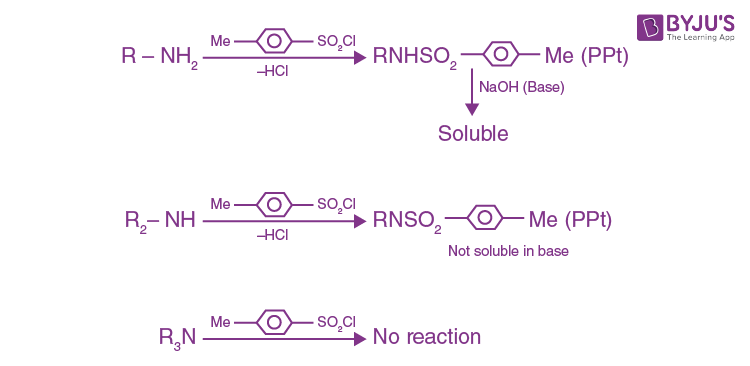Solution:Question 17. Match List-I with List-II

 List-I List-II (a) Haematite (i) Al2O3. xH2O (b) Bauxite (ii) Fe2O3 (c) Magnetite (iii) CuCO3. Cu(OH)2 (d) Malachite (iv) Fe3O4

Choose the correct answer from the options given below:

1. a. (a)-(ii), (b)-(iii), (c)-(i), (d)-(iv)
2. b. (a)-(iv), (b)-(i), (c)-(ii), (d)-(iii)
3. c. (a)-(i), (b)-(iii), (c)-(ii), (d)-(iv)/span> d. (a)-(ii), (b)-(i), (c)-(iv), (d)-(iii)

Solution:

Fact

Question 18. The set that represents the pair of neutral oxides of nitrogen is:

1. a. NO and N2O
2. b. NO and NO2
3. c. N2O and NO2
4. d. N2O and N2O3

Solution:

NO and N2O are neutral oxides and N2O3, NO2 and N2O5 are acidic oxides.

Question 19. Nitrogen can be estimated by Kjeldahl’s method for which of the following compound?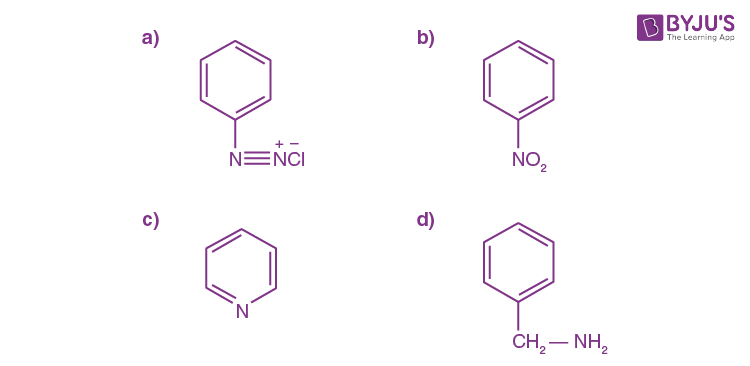Solution:

If the nitrogen atom is a part of the ring or in the form of the diazonium or NO2, then it is not estimated with Kjeldahl's method.

Question 20. One of the by-products formed during the recovery of NH3 from Solvay process is:

1. a. NaHCO3
2. b. Ca(OH)2
3. c. CaCl2
4. d. NH4Cl

Solution:

Recovery of NH3

2NH4Cl + Ca(OH)2 → CaCl2 + 2NH3 + 2H2O

Byproduct

SECTION – B

Question 21. The reaction 2A + B2→ 2AB is an elementary reaction. For a certain quantity of reactants, if the volume of the reaction vessel is reduced by a factor of 3, the rate of the reaction increases by a factor of …………………. (Round off to the Nearest Integer).

Solution:

For elementary reaction,

Rate of reaction = K[A]2 [B2]

Initial rate =

$$K\left ( \frac{n_{A}}{v_{o}} \right )^{2}\left ( \frac{n_{B}}{v_{o}} \right )$$

Final rate =

$$K\left ( \frac{n_{A}}{v_{o}/3} \right )^{2}\left ( \frac{n_{B}}{v_{o}/3} \right ) = 27K\left ( \frac{n_{A}}{v_{o}} \right )\left ( \frac{n_{B}}{v_{o}} \right )$$

Final rate = 27 × Initial rate

Question 22. In the ground state of atomic Fe (Z = 26), the spin-only magnetic moment is ……………..x 10-1 BM. (Round off to the Nearest Integer).

[Given: √3 = 1.73, √2 = 1.41]

Solution:

Fe: 1s2 2s2 2p6 3s2 3p6 3d6 4s2

In 3d6, no. of unpaired e = 4

Spin only magnetic moment = √n(n+2) BM

= √4(4+2)

= √24

= 4.9

= 49 × 10–1

Question 23. Consider the below-given reaction. The percentage yield of an amide product is ………………. (Round off to the Nearest Integer). [Given: Atomic mass: C: 12.0 u, H : 1.0 u, N : 14.0, O : 16.0 u, Cl : 35.5 u]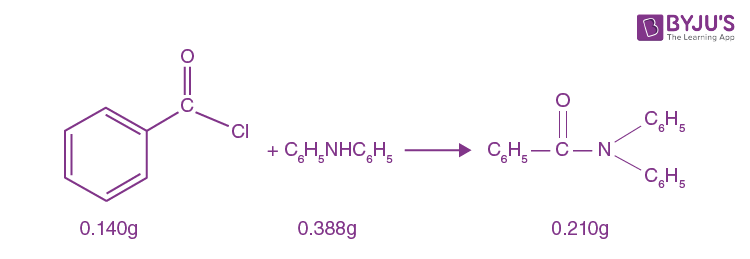Solution: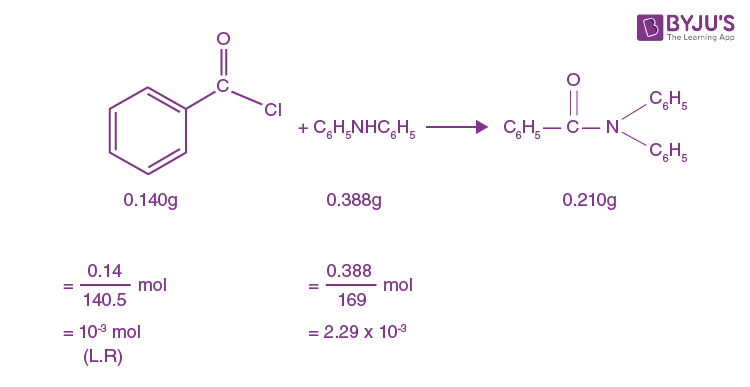Stoichiometric moles of amide = 10–3 mol

Actual moles of amide = 7.69 × 10–4 mol

% yield =

$$\frac{7.69\times 10^{-4}\times 100}{10^{-3}}$$

= 76.9%

≃ 77%

Question 24. On complete reaction of FeCl3 with oxalic acid in an aqueous solution containing KOH, resulted in the formation of product A. The secondary valency of Fe in the product A is …………..(Round off to the Nearest Integer)

Solution: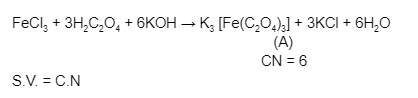Question 25. Consider the reaction N2O4 (g) ⇌ 2NO2 (g). The temperature at which KC = 20.4 and KP = 600.1, is …………………. K. (Round off to the Nearest Integer). [Assume all gases are ideal and R = 0.0831 L bar K-1 mol-1]

Solution:

KP = KC (RT)∆ng, ∆ng= 1 (for given reaction)

600.1 = 20.4 (RT)1

T ≈ 354 K

Question 26. A KCl solution of conductivity 0.14 S m-1 shows a resistance of 4.19 Ω in a conductivity cell. If the same cell is filled with an HCl solution, the resistance drops 1.03 Ω. The conductivity of the HCl solution is ……………….. x 10-2 S m-1. (Round off to the Nearest Integer).

Solution:

For KCl solution,

$$R = \left (\frac{1}{K} \right )\left (\frac{l}{A} \right ) \Rightarrow \frac{l}{A} = R \times K = 4.19\times0.14$$

= 0.58 ohm

For HCl solution,

$$R = \left (\frac{1}{K} \right )\left (\frac{l}{A} \right )$$

$$K = \left (\frac{l/A}{R} \right ) = \frac{0.58}{1.03}=0.56=56\times 10^{-2} \: Sm^{-1}$$

= 56

Question 27. A 1 molal K4Fe(CN)6 solution has a degree of dissociation of 0.4. Its boiling point is equal to that of another solution which contains 18.1 weight per cent of a nonelectrolyte solute A. The molar mass of A is …………… g/mol. (Round off to the Nearest Integer).

Solution:

Since boiling point is the same, elevation in boiling point is also the same for both solutions.

$$(\Delta T_{B})_{K_{4}[Fe(CN)_{6}]} = (\Delta T_{B})_{A}$$

$$(ik_{B}m)_{K_{4}[Fe(CN)_{6}]} = (ik_{B}m)_{A}$$

$$(1+4\alpha)\times 1 = \frac{1\times \frac{18.1}{M}\times 1000}{100-18.1}$$

$$2.6 = \frac{18.1}{M}\times \frac{1000}{81.9}$$

M = 85

Question 28. The number of chlorine atoms in 20 mL of chlorine gas at STP is ………1021. (Round off to the Nearest Integer).

[Assume chlorine is an ideal gas at STP

R=0.083 L bar mol-1 K-1, NA = 6.023 x 1023]

Solution:

$$n = \frac{PV}{RT}=\frac{1\times20 \times10^{-3} }{0.083\times 273}$$

$$Number\: of\: atoms = \frac{1\times20 \times10^{-3} }{0.083\times 273} \times2 \times6.023 \times10^{23}$$

= 1.06 × 1021

Question 29. KBr is doped with 10-5 mole per cent of SrBr2. The number of cationic vacancies in 1 g of KBr crystal is ……… 1014. (Round off to the Nearest Integer).

[Atomic Mass: K = 39.1 u, Br = 79.9 u, NA = 6.023 x 1023]

Solution:

For every Sr+2 ion, 1 cationic vacancy is created. Hence, no. of Sr+2 ion = Number of cationic vacancies

Since the mole percentage of SrBr2 dopped is 10–5 to that of total moles of KBr.

Question 30. The total number of C–C sigma bond/s in mesityl oxide (C6H10O) is …… (Round off to the Nearest Integer).

Solution: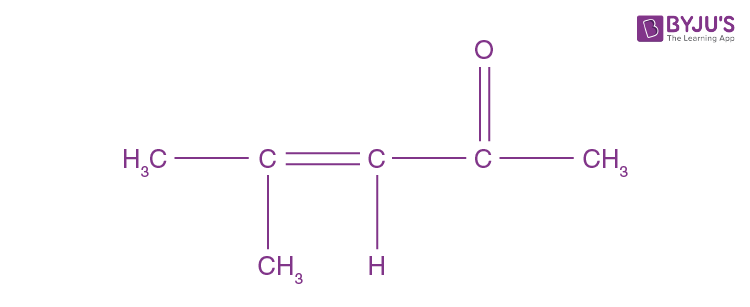### JEE Main 2021 Chemistry Paper March 17 Shift 2